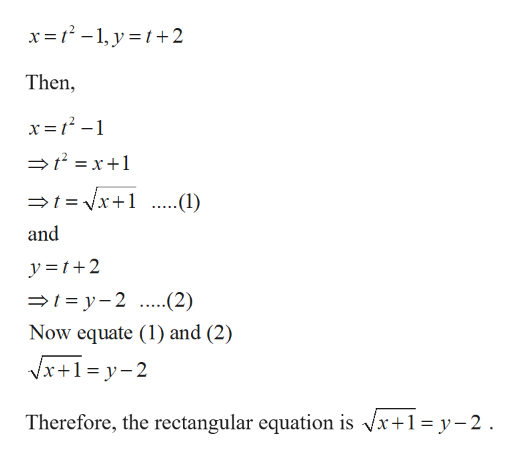# Graph the parametric graph and find the corresponding rectangular equation by eliminating the parameter. x=t^2-1,y=t+2.

Question
16 views

Graph the parametric graph and find the corresponding rectangular equation by eliminating the parameter. x=t^2-1,y=t+2.

check_circle

Step 1

Given,

...help_outlineImage Transcriptionclosex=1² - 1, y =t+2 Then, x= 1? -1 =t = x+1 =t= Vx+1 ...(1) ...... and y =t+2 =1 = y- 2 ...(2) Now equate (1) and (2) Vx+1= y-2 Therefore, the rectangular equation is Vx+1=y-2. fullscreen

### Want to see the full answer?

See Solution

#### Want to see this answer and more?

Solutions are written by subject experts who are available 24/7. Questions are typically answered within 1 hour.*

See Solution
*Response times may vary by subject and question.
Tagged in

### Calculus